# AI & Statistics 2005

## Abstracts of Papers

A Uniform Convergence Bound for the Area Under the ROC Curve

Shivani Agarwal, Sariel Har-Peled and Dan Roth

The area under the ROC curve (AUC) has been advocated as an evaluation criterion for the bipartite ranking problem. We study uniform convergence properties of the AUC; in particular, we derive a distribution-free uniform convergence bound for the AUC which serves to bound the expected accuracy of a learned ranking function in terms of its empirical AUC on the training sequence from which it is learned. Our bound is expressed in terms of a new set of combinatorial parameters that we term the bipartite rank-shatter coefficients; these play the same role in our result as do the standard VC-dimension related shatter coefficients (also known as the growth function) in uniform convergence results for the classification error rate. A comparison of our result with a recent uniform convergence result derived by Freund et al. (2003) for a quantity closely related to the AUC shows that the bound provided by our result can be considerably tighter.

On the Path to an Ideal ROC Curve: Considering Cost Asymmetry in Learning Classifiers

Francis Bach, David Heckerman and Eric Horvitz

Receiver Operating Characteristic (ROC) curves are a standard way to display the performance of a set of binary classifiers for all feasible ratios of the costs associated with false positives and false negatives. For linear classifiers, the set of classifiers is typically obtained by training once, holding constant the estimated slope and then varying the intercept to obtain a parameterized set of classifiers whose performances can be plotted in the ROC plane. In this paper, we consider the alternative of varying the asymmetry of the cost function used for training. We show that the ROC curve obtained by varying the intercept and the asymmetry — and hence the slope — always outperforms the ROC curve obtained by varying only the intercept. In addition, we present a path-following algorithm for the support vector machine (SVM) that can compute efficiently the entire ROC curve, that has the same computational properties as training a single classifier. Finally, we provide a theoretical analysis of the relationship between the asymmetric cost model assumed when training a classifier and the cost model assumed in applying the classifier. In particular, we show that the mismatch between the step function used for testing and its convex upper bounds usually used for training leads to a provable and quantifiable difference around extreme asymmetries.

On Manifold Regularization

Misha Belkin, Partha Niyogi and Vikas Sindhwani

We propose a family of learning algorithms based on a new form of regularization that allows us to exploit the geometry of the marginal distribution. We focus on a semi-supervised framework that incorporates labeled and unlabeled data in a general-purpose learner. Some transductive graph learning algorithms and standard methods including Support Vector Machines and Regularized Least Squares can be obtained as special cases. We utilize properties of Reproducing Kernel Hilbert spaces to prove new Representer theorems that provide theoretical basis for the algorithms. As a result (in contrast to purely graph based approaches) we obtain a natural out-of-sample extension to novel examples and are thus able to handle both transductive and truly semi-supervised settings. We present experimental evidence suggesting that our semi-supervised algorithms are able to use unlabeled data effectively. In the absence of labeled examples, our framework gives rise to a regularized form of spectral clustering with an out-of-sample extension.

Distributed Latent Variable Models of Lexical Co-occurrences

John Blitzer, Amir Globerson and Fernando Pereira

Low-dimensional representations for lexical co-occurrence data have become increasingly important in alleviating the sparse data problem inherent in natural language processing tasks. This work presents a distributed latent variable model for inducing these low-dimensional representations. The model takes inspiration from both connectionist language models (Bengio et al. 2003, Xu et al. 2003) and (Saul and Pereira 1997, Hofmann and Puzicha 1998). We give results which show that the new model significantly improves both bigram and trigram models.

On Contrastive Divergence Learning

Miguel Á. Carreira-Perpiñán and Geoffrey Hinton

Maximum-likelihood (ML) learning of Markov random fields is challenging because it requires estimates of averages that have an exponential number of terms. Markov chain Monte Carlo methods typically take a long time to converge on unbiased estimates, but Hinton (2002) showed that if the Markov chain is only run for a few steps, the learning can still work well and it approximately minimizes a different function called “contrastive divergence” (CD). CD learning has been successfully applied to various types of random fields. Here, we study the properties of CD learning and show that it provides biased estimates in general, but that the bias is typically very small. Fast CD learning can therefore be used to get close to an ML solution and slow ML learning can then be used to fine-tune the CD solution.

OOBN for Forensic Identification through Searching a DNA profiles’ Database

In this paper we evaluate forensic identification hypotheses conditionally to the characteristics observed both on a crime sample and on individuals contained in a database. First we solve the problem via a computational efficient Bayesian Network obtained by transforming some recognized conditional specific independencies into conditional independencies. Then we propose an Object Oriented Bayesian Network representation, first considering a generic characteristic, then inheritable DNA traits.In this respect we show how to use the Object Oriented Bayesian Network to evaluate hypotheses concerning the possibility that some unobserved individuals, genetically related to the individuals profiled in the database, are the donors of the crime sample.

Active Learning for Parzen Window Classifier

Olivier Chapelle

The problem of active learning is approached in this paper by minimizing directly an estimate of the expected test error. The main difficulty in this “optimal” strategy is that output probabilities need to be estimated accurately. We suggest here different methods for estimating those efficiently. In this context, the Parzen window classifier is considered because it is both simple and probabilistic. The analysis of experimental results highlights that regularization is a key ingredient for this strategy.

Semi-Supervised Classification by Low Density Separation

Olivier Chapelle and Alexander Zien

We believe that the cluster assumption is key to successful semi-supervised learning. Based on this, we propose three semi-supervised algorithms: 1. deriving graph-based distances that emphazise low density regions between clusters, followed by training a standard SVM; 2. optimizing the Transductive SVM objective function, which places the decision boundary in low density regions, by gradient descent; 3. combining the first two to make maximum use of the cluster assumption. We compare with state of the art algorithms and demonstrate superior accuracy for the latter two methods.

Learning spectral graph segmentation

Timothée Cour, Nicolas Gogin and Jianbo Shi

We present a general graph learning algorithm for spectral graph partitioning, that allows direct supervised learning of graph structures using hand labeled training examples. The learning algorithm is based on gradient descent in the space of all feasible graph weights. Computation of the gradient involves finding the derivatives of eigenvectors with respect to the graph weight matrix. We show the derivatives of eigenvectors exist and can be computed in an exact analytical form using the theory of implicit functions. Furthermore, we show for a simple case, the gradient converges exponentially fast. In the image segmentation domain, we demonstrate how to encode top-down high level object prior in a bottom-up shape detection process.

A Graphical Model for Simultaneous Partitioning and Labeling

Philip J. Cowans and Martin Szummer

In this work we develop a graphical model for describing probability distributions over labeled partitions of an undirected graph which are conditioned on observed data. We show how to efficiently perform exact inference in these models, by exploiting the structure of the graph and adapting the sum-product and max-product algorithms. We demonstrate our approach on the task of segmenting and labeling hand-drawn ink fragments, and show that a significant performance increase is obtained by labeling and partitioning simultaneously.

Restructuring Dynamic Causal Systems in Equilibrium

Denver Dash

In this paper I consider general obstacles to the recovery of a causal system from its probability distribution. I argue that most of the well-known problems with this task belong in the class of what I call degenerate causal systems. I then consider the task of discovering causality of dynamic systems that have passed through one or more equilibrium points, and show that these systems present a challenge to causal discovery that is fundamentally different from degeneracy. To make this comparison, I consider two operators that are used to transform causal models. The first is the well-known Do operator for modeling manipulation, and the second is the Equilibration operator for modeling a dynamic system that has achieved equilibrium. I consider a set of questions regarding the commutability of these operators i.e., whether or not an equilibrated-manipulated model is necessarily equal to the corresponding manipulated-equilibrated model, and I explore the implications of that commutability on the practice of causal discovery. I provide empirical results showing that (a) these two operators sometimes, but not always, commute, and (b) the manipulated-equilibrated model is the correct one under a common interpretation of manipulation on dynamic systems. I argue that these results have strong implications for causal discovery from equilibrium data.

Probability and Statistics in the Law

Philip Dawid

The field of legal reasoning is full of logical subtleties and probabilistic pitfalls. I survey a number of these, pointing out some of the problems and ambiguities, and various attempts to deal with them. Some celebrated court cases are used for illustration.

Efficient Non-Parametric Function Induction in Semi-Supervised Learning

Olivier Delalleau, Yoshua Bengio and Nicolas Le Roux

There has been an increase of interest for semi-supervised learning recently, because of the many datasets with large amounts of unlabeled examples and only a few labeled ones. This paper follows up on proposed non-parametric algorithms which provide an estimated continuous label for the given unlabeled examples. First, it extends them to function induction algorithms that minimize a regularization criterion applied to an out-of-sample example, and happen to have the form of Parzen windows regressors. This allows to predict test labels without solving again a linear system of dimension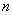(the number of unlabeled and labeled training examples), which can cost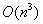. Second, this function induction procedure gives rise to an efficient approximation of the training process, reducing the linear system to be solved to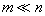unknowns, using only a subset of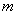examples. An improvement of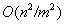in time can thus be obtained. Comparative experiments are presented, showing the good performance of the induction formula and approximation algorithm.

Structured Variational Inference Procedures and their Realizations

Dan Geiger and Chris Meek

We describe and prove the convergence of several algorithms for approximate structured variational inference. We discuss the computation cost of these algorithms and describe their relationship to the mean-field and generalized-mean-field variational approaches and other structured variational methods.

Kernel Constrained Covariance for Dependence Measurement

Arthur Gretton, Alexander Smola, Olivier Bousquet, Ralf Herbrich, Andrei Belitski, Mark Augath, Yusuke Murayama, Jon Pauls, Bernhard Schölkopf and Nikos Logothetis

We discuss reproducing kernel Hilbert space (RKHS)-based measures of statistical dependence, with emphasis on constrained covariance (COCO), a novel criterion to test dependence of random variables. We show that COCO is a test for independence if and only if the associated RKHSs are universal. That said, no independence test exists that can distinguish dependent and independent random variables in all circumstances. Dependent random variables can result in a COCO which is arbitrarily close to zero when the source densities are highly non-smooth. All current kernel-based independence tests share this behaviour. We demonstrate exponential convergence between the population and empirical COCO. Finally, we use COCO as a measure of joint neural activity between voxels in MRI recordings of the macaque monkey, and compare the results to the mutual information and the correlation. We also show the effect of removing breathing artefacts from the MRI recording.

Semisupervised alignment of manifolds

Jihun Ham, Daniel Lee and Lawrence Saul

In this paper, we study a family of semisupervised learning algorithms for “aligning” different data sets that are characterized by the same underlying manifold. The optimizations of these algorithms are based on graphs that provide a discretized approximation to the manifold. Partial alignments of the data sets—obtained from prior knowledge of their manifold structure or from pairwise correspondences of subsets of labeled examples—are completed by integrating supervised signals with unsupervised frameworks for manifold learning. As an illustration of this semisupervised setting, we show how to learn mappings between different data sets of images that are parameterized by the same underlying modes of variability (e.g., pose and viewing angle). The curse of dimensionality in these problems is overcome by exploiting the low dimensional structure of image manifolds.

Learning Causally Linked Markov Random Fields

Geoffrey Hinton, Simon Osindero and Kejie Bao

We describe a learning procedure for a generative model that contains a hidden Markov Random Field (MRF) which has directed connections to the observable variables. The learning procedure uses a variational approximation for the posterior distribution over the hidden variables. Despite the intractable partition function of the MRF, the weights on the directed connections and the variational approximation itself can be learned by maximizing a lower bound on the log probability of the observed data. The parameters of the MRF are learned by using the mean field version of contrastive divergence . We show that this hybrid model simultaneously learns parts of objects and their inter-relationships from intensity images. We discuss the extension to multiple MRF’s linked into in a chain graph by directed connections.

Hilbertian Metrics and Positive Definite Kernels on Probability Measures

Matthias Hein and Olivier Bousquet

We investigate the problem of defining Hilbertian metrics resp. positive definite kernels on probability measures, continuing the work in .This type of kernels has shown very good results in text classification and has a wide range of possible applications. In this paper we extend the two-parameter family of Hilbertian metrics of Topsøe such that it now includes all commonly used Hilbertian metrics on probability measures. This allows us to do model selection among these metrics in an elegant and unified way. Second we investigate further our approach to incorporate similarity information of the probability space into the kernel. The analysis provides a better understanding of these kernels and gives in some cases a more efficient way to compute them. Finally we compare all proposed kernels in two text and two image classification problems.

Fast Non-Parametric Bayesian Inference on Infinite Trees

Marcus Hutter

Given i.i.d. data from an unknown distribution, we consider the problem of predicting future items. An adaptive way to estimate the probability density is to recursively subdivide the domain to an appropriate data-dependent granularity. A Bayesian would assign a data-independent prior probability to "subdivide", which leads to a prior over infinite(ly many) trees. We derive an exact, fast, and simple inference algorithm for such a prior, for the data evidence, the predictive distribution, the effective model dimension, and other quantities.

Restricted concentration models – graphical Gaussian models with concentration parameters restricted to being equal

Søren Højsgaard and Steffen Lauritzen

In this paper we introduce restricted concentration models (RCMs) as a class of graphical models for the multivariate Gaussian distribution in which some elements of the concentration matrix are restricted to being identical is introduced. An estimation algorithm for RCMs, which is guaranteed to converge to the maximum likelihood estimate, is presented. Model selection is briefly discussed and a practical example is given.

Fast maximum a-posteriori inference on Monte Carlo state spaces

Mike Klaas, Dustin Lang and Nando de Freitas

Many important algorithms for statistical inference can be expressed as a weighted max-kernel search problem. This is the case with the Viterbi algorithm for HMMs, message construction in maximum a posteriori  BP (max-BP), as well as certain particle-smoothing algorithms. Previous work has focused on reducing the cost of this procedure in discrete regular grids . Monte- Carlo state spaces, which are vital for high-dimensional inference, cannot be handled by these techniques. We present a novel dual-tree based algorithm that is appliable to a wide range of kernels and shows substantial performance gains over naïve computation.

Generative Model for Layers of Appearance and Deformation

Anitha Kannan, Nebojsa Jojic and Brendan Frey

We are interested in learning generative models of objects that can be used in wide range of tasks such as video summarization, image segmentation and frame interpolation. Learning object-based appearance/shape models and estimating motion fields (deformation field) are highly interdependent problems. At the extreme, all motions can be represented as an excessively large set of appearance exemplars. However, a more efficient representation of a video sequence would save on frame description if it described the motion from the previous frame instead. The extreme in this direction is also problematic as there are usually causes of appearance variability other than motion. The flexible sprite model (Jojic and Frey, 2001) illustrates the benefits of joint modelling of motion, shape and appearance using very simple models. The advantage of such a model is that each part of the model tries to capture some of the variability in the data until all the variability is decomposed and explained through either appearance, shape or transformation changes. Yet, the set of motions modelled is very limited, and the residual motion is simply captured in the variance maps of the sprites. In this paper, we develop a better balance between the transformation and appearance model by explicitly modelling arbitrary large, non-uniform motion.

Toward Question-Asking Machines: The Logic of Questions and the Inquiry Calculus

Kevin Knuth

For over a century, the study of logic has focused on the algebra of logical statements. This work, first performed by George Boole, has led to the development of modern computers, and was shown by Richard T. Cox to be the foundation of Bayesian inference. Meanwhile the logic of questions has been much neglected. For our computing machines to be truly intelligent, they need to be able to ask relevant questions. In this paper I will show how the Boolean lattice of logical statements gives rise to the free distributive lattice of questions thus defining their algebra. Furthermore, there exists a quantity analogous to probability, called relevance, which quantifies the degree to which one question answers another. I will show that relevance is not only a natural generalization of information theory, but also forms its foundation.

Convergent tree-reweighted message passing for energy minimization

Tree-reweighted max-product message passing (TRW) is an algorithm for energy minimization introduced recently by Wainwright et al. . It shares some similarities with Pearl’s loopy belief propagation. TRW was inspired by a problem of maximizing a lower bound on the energy. However, the algorithm is not guaranteed to increase this bound - it may actually go down. In addition, TRW does not always converge. We develop a modification of this algorithm which we call sequential tree-reweighted message passing. Its main property is that the bound is guaranteed not to decrease. We also give a weak tree agreement condition which characterizes local maxima of the bound with respect to TRW algorithms. We prove that our algorithm has a limit point that achieves weak tree agreement. Experimental results demonstrate that on certain synthetic and real problems our algorithm outperforms both the ordinary belief propagation and tree-reweighted algorithm .

Instrumental variable tests for Directed Acyclic Graph Models

Manabu Kuroki and Zhihong Cai

Consider a case where cause-effect relationships among variables can be described as a directed acyclic graph model. The instrumental variable (IV) method is well known as a powerful tool for inferring the total effect of a treatment variable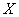on a response variable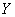, even if there exist unobserved confounders betweenand. This paper proposes a criterion to search for covariates which satisfy the IV conditions in linear structural equation models. In addition, it is shown that our criterion is applicable to the case where all variables in a directed acyclic graph are discrete. The results of this paper provide a statistical justification for testing whether the statistical data is generated by a model involving IVs.

Estimating Class Membership Probabilities using Classifier Learners

We present an algorithm, “Probing”, which reduces learning an estimator of class probability membership to learning binary classifiers. The reduction comes with a theoretical guarantee: a small error rate for binary classification implies accurate estimation of class membership probabilities. We tested our reduction on several datasets with several classifier learning algorithms. The results show strong performance as compared to other common methods for obtaining class membership probability estimates from classifiers.

Loss Functions for Discriminative Training of Energy-Based Models

Yann LeCun and Fu Jie Huang

Probabilistic graphical models associate a probability to each configuration of the relevant variables. Energy-based models  (EBM) associate an energy to those configurations, eliminating the need for proper normalization of probability distributions. Making a decision (an inference) with an EBM consists in comparing the energies associated with various configurations of the variable to be predicted, and choosing the one with the smallest energy. Such systems must be trained discriminatively to associate low energies to the desired configurations and higher energies to undesired configurations. A wide variety of loss function can be used for this purpose. We give sufficient conditions that a loss function should satisfy so that its minimization will cause the system to approach to desired behavior. We give many specific examples of suitable loss functions, and show an application to object recognition in images.

Probabilistic Soft Interventions in Conditional Gaussian Networks

Florian Markowetz, Steffen Grossmann, and Rainer Spang

We introduce a general concept of probabilistic interventions in Bayesian networks. This generalizes deterministic interventions, which fix nodes to certain states. We propose “pushing” variables in the direction of target states without fixing them. We formalize this idea in a Bayesian framework based on Conditional Gaussian networks.

Unsupervised Learning with Non-Ignorable Missing Data

Benjamin M. Marlin, Sam T. Roweis and Richard S. Zemel

In this paper we explore the topic of unsupervised learning in the presence of non-ignorable missing data with an unknown missing data mechanism. We discuss several classes of missing data mechanisms for categorical data and develop learning and inference methods for two specific models. We present empirical results using synthetic data which show that these algorithms can recover both the unknown selection model parameters and the underlying data model parameters to a high degree of accuracy. We also apply the algorithms to real data from the domain of collaborative filtering, and report initial results.

Regularized spectral learning

Marina Meila, Susan Shortreed and Liang Xu

Spectral clustering is a technique for finding groups in data consisting of similarities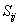between pairs of points. We approach the problem of learning the similarity as a function of other observed features, in order to optimize spectral clustering results on future data. This paper formulates a new objective for learning in spectral clustering, that balances a clustering accuracy term, the gap, and a stability term, the eigengap with the later in the role of a regularizer. We derive an algorithm to optimize this objective, and semiautomatic methods to chose the optimal regularization. Preliminary experiments confirm the validity of the approach.

Approximate Inference for Infinite Contingent Bayesian Networks

Brian Milch, Bhaskara Marthi, David Sontag, Stuart Russell, Daniel L. Ong and Andrey Kolobov

In many practical problems — from tracking aircraft based on radar data to building a bibliographic database based on citation lists — we want to reason about an unbounded number of unseen objects with unknown relations among them. Bayesian networks, which define a fixed dependency structure on a finite set of variables, are not the ideal representation language for this task. This paper introduces contingent Bayesian networks (CBNs), which represent uncertainty about dependencies by labeling each edge with a condition under which it is active. A CBN may contain cycles and have infinitely many variables. Nevertheless, we give general conditions under which such a CBN defines a unique joint distribution over its variables. We also present a likelihood weighting algorithm that performs approximate inference in finite time per sampling step on any CBN that satisfies these conditions.

Hierarchical Probabilistic Neural Network Language Model

Frederic Morin and Yoshua Bengio

In recent years, variants of a neural network architecture for statistical language modeling have been proposed and successfully applied, e.g. in the language modeling component of speech recognizers. The main advantage of these architectures is that they learn an embedding for words (or other symbols) in a continuous space that helps to smooth the language model and provide good generalization even when the number of training examples is insufficient. However, these models are extremely slow in comparison to the more commonly used n-gram models, both for training and recognition. As an alternative to an importance sampling method proposed to speed-up training, we introduce a hierarchical decomposition of the conditional probabilities that yields a speed-up of about 200 both during training and recognition. The hierarchical decomposition is a binary hierarchical clustering constrained by the prior knowledge extracted from the WordNet semantic hierarchy.

Greedy Spectral Embedding

Marie Ouimet and Yoshua Bengio

Spectral dimensionality reduction methods and spectral clustering methods require computation of the principal eigenvectors of an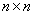matrix whereis the number of examples. Following up on previously proposed techniques to speed-up kernel methods by focusing on a subset ofexamples, we study a greedy selection procedure for this subset, based on the feature-space distance between a candidate example and the span of the previously chosen ones. In the case of kernel PCA or spectral clustering this reduces computation to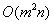. For the same computational complexity, we can also compute the feature space projection of the non-selected examples on the subspace spanned by the selected examples, to estimate the embedding function based on all the data, which yields considerably better estimation of the embedding function. This algorithm can be formulated in an online setting and we can bound the error on the approximation of the Gram matrix.

FastMap, MetricMap, and Landmark MDS are all Nystrom Algorithms

John Platt

This paper unifies the mathematical foundation of three multidimensional scaling algorithms: FastMap, MetricMap, and Landmark MDS (LMDS). All three algorithms are based on the Nyström approximation of the eigenvectors and eigenvalues of a matrix. LMDS is applies the basic Nyström approximation, while FastMap and MetricMap use generalizations of Nyström, including deflation and using more points to establish an embedding. Empirical experiments on the Reuters and Corel Image Features data sets show that the basic Nyström approximation outperforms these generalizations: LMDS is more accurate than FastMap and MetricMap with roughly the same computation and can become even more accurate if allowed to be slower.

Bayesian Conditional Random Fields

Yuan Qi, Martin Szummer and Tom Minka

We propose Bayesian Conditional Random Fields (BCRFs) for classifying interdependent and structured data, such as sequences, images or webs. BCRFs are a Bayesian approach to training and inference with conditional random fields, which were previously trained by maximizing likelihood (ML) (Lafferty et al., 2001). Our framework eliminates the problem of overfitting, and offers the full advantages of a Bayesian treatment. Unlike the ML approach,we estimate the posterior distribution of the model parameters during training, and average over this posterior during inference. We apply an extension of EP method, the power EP method, to incorporate the partition function. For algorithmic stability and accuracy, we flatten the approximation structures to avoid two-level approximations. Finally, BCRFs use low-rank matrix computation for computational efficiency. We demonstrate the superior prediction accuracy of BCRFs over conditional random fields trained with ML or MAP on synthetic and real datasets.

Poisson-Networks: A Model for Structured Poisson Processes

Shyamsundar Rajaram, Graepel Thore and Ralf Herbrich

Modelling structured multivariate point process data has wide ranging applications like understanding neural activity, developing faster file access systems and learning dependencies among servers in large networks. In this paper, we develop the Poisson network model for representing multivariate structured Poisson processes. In our model each node of the network represents a Poisson process. The novelty of our work is that waiting times of a process are modelled by an exponential distribution with a piecewise constant rate function that depends on the event counts of its parents in the network in a generalised linear way. Our choice of model allows to perform exact sampling from arbitrary structures. We adopt a Bayesian approach for learning the network structure. Further, we discuss fixed point and sampling based approximations for performing inference of rate functions in Poisson networks.

Deformable Spectrograms

Manuel Reyes-Gomez, Nebojsa Jojic and Daniel Ellis

Speech and other natural sounds show high temporal correlation and smooth spectral evolution punctuated by a few, irregular and abrupt changes. In a conventional Hidden Markov Model (HMM), such structure is represented weakly and indirectly through transitions between explicit states representing ‘steps’ along such smooth changes. It would be more efficient and informative to model successive spectra as transformations of their immediate predecessors, and we present a model which focuses on local deformations of adjacent bins in a time-frequency surface to explain an observed sound, using explicit representation only for those bins that cannot be predicted from their context. We further decompose the log-spectrum into two additive layers, which are able to separately explain and model the evolution of the harmonic excitation, and formant filtering of speech and similar sounds. Smooth deformations are modeled with hidden transformation variables in both layers, using Markov Random fields (MRFs) with overlapping subwindows as observations; inference is efficiently performed via loopy belief propagation. The model can fill-in deleted time-frequency cells without any signal model, and an entire signal can be compactly represented with a few specific states along with the deformation maps for both layers. We discuss several possible applications for this new model, including source separation.

Variational Speech Separation of More Sources than Mixtures

Steven J. Rennie, Kannan Achan, Brendan J. Frey and Parham Aarabi

We present a novel structured variational inference algorithm for probabilistic speech separation. The algorithm is built upon a new generative probability model of speech production and mixing in the full spectral domain, that utilizes a detailed probability model of speech trained in the magnitude spectral domain, and the position ensemble of the underlying sources as a natural, low-dimensional parameterization of the mixing process. The algorithm is able to produce high quality estimates of the underlying source configurations, even when there are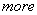underlying sources than available microphone recordings. Spectral phase estimates of all underlying speakers are automatically recovered by the algorithm, facilitating the direct transformation of the obtained source estimates into the time domain, to yield speech signals of high perceptual quality.

Learning Bayesian Network Models from Incomplete Data using Importance Sampling

We propose a Bayesian approach to learning Bayesian network models from incomplete data. The objective is to obtain the posterior distribution of models, given the observed part of the data. We describe a new algorithm, called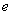MC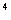, to simulate draws from this posterior distribution. One of the new ideas in our algorithm is to use importance sampling to approximate the posterior distribution of models given the observed data and the current imputation model. The importance sampler is constructed by defining an approximate predictive distribution for the unobserved part of the data. In this way existing (heuristic) imputation methods can be used that don’t require exact inference for generating imputations.

We illustrateMCby its application to modeling the risk factors of coronary heart disease. In the experiments we consider different missing data mechanisms and different fractions of missing data.

On the Behavior of MDL Denoising

Teemu Roos, Petri Myllymäki and Henry Tirri

We consider wavelet denoising based on minimum description length (MDL) principle. The derivation of an MDL denoising criterion proposed by Rissanen involves a renormalization whose effect on the resulting method has not been well understood so far. By inspecting the behavior of the method we obtain a characterization of its domain of applicability: good performance in the low variance regime but over-fitting in the high variance regime. We also describe unexpected behavior in the theoretical situation where the observed signal is pure noise. An interpretation for the renormalization is given which explains both the empirical and theoretical findings. For practitioners we point out two technical pitfalls and ways to avoid them. Further, we give guidelines for constructing improved MDL denoising

Focused Inference

Romer Rosales and Tommi Jaakkola

We develop a method similar to variable elimination for computing approximate marginals in graphical models. An underlying notion in this method is that it is not always necessary to compute marginals over all the variables in the graph, but focus on a few variables of interest. The Focused Inference (FI) algorithm introduced reduces the original distribution to a simpler one over the variables of interest. This is done in an iterative manner where in each step the operations are guided by (local) optimality properties. We exemplify various properties of the focused inference algorithm and compare it with other methods. Numerical simulation indicates that FI outperform the competing methods.

Kernel Methods for Missing Variables

Alex J. Smola, S. V. N. Vishwanathan and Thomas Hofmann

We present methods for dealing with missing variables in the context of Gaussian Processes and Support Vector Machines. This solves an important problem which has largely been ignored by kernel methods: How to systematically deal with incomplete data? Our method can also be applied to problems with partially observed labels as well as to the transductive setting where we view the labels as missing data. Our approach relies on casting kernel methods as an estimation problem in exponential families. Hence, estimation with missing variables becomes a problem of computing marginal distributions, and finding efficient optimization methods. To that extent we propose an optimization scheme which extends the Concave Convex Procedure (CCP) of Yuille and Rangarajan, and present a simplified and intuitive proof of its convergence. We show how our algorithm can be specialized to various cases in order to efficiently solve the optimization problems that arise. Encouraging preliminary experimental results on the USPS dataset are also presented.

Semiparametric latent factor models

Yee Whye Teh, Matthias Seeger and Michael I. Jordan

We propose a semiparametric model for regression problems involving multiple response variables. The model makes use of a set of Gaussian processes that are linearly mixed to capture dependencies that may exist among the response variables. We propose an efficient approximate inference scheme for this semiparametric model whose complexity is linear in the number of training data points. We present experimental results in the domain of multi-joint robot arm dynamics.

Efficient Gradient Computation for Conditional Gaussian Models

Bo Thiesson and Chris Meek

We introduce Recursive Exponential Mixed Models (REMMs) and derive the gradient of the parameters for the incomplete-data likelihood. We demonstrate how one can use probabilistic inference in Conditional Gaussian (CG) graphical models, a special case of REMMs, to compute the gradient for a CG model. We also demonstrate that this approach can yield simple and effective algorithms for computing the gradient for models with tied parameters and illustrate this approach on stochastic ARMA models.

Very Large SVM Training using Core Vector Machines

Ivor Tsang, James Kwok and Pak-Ming Cheung

Standard SVM training has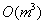time and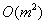space complexities, whereis the training set size. In this paper, we scale up kernel methods by exploiting the “approximateness” in practical SVM implementations. We formulate many kernel methods as equivalent minimum enclosing ball problems in computational geometry, and then obtain provably approximately optimal solutions efficiently with the use of core-sets. Our proposed Core Vector Machine (CVM) algorithm has a time complexity that is linear inand a space complexity that is independent of. Experiments on large toy and real-world data sets demonstrate that the CVM is much faster and can handle much larger data sets than existing scale-up methods. In particular, on our PC with only 512M RAM, the CVM with Gaussian kernel can process the checkerboard data set with 1 million points in less than 13 seconds.

Streaming Feature Selection using IIC

Lyle H. Ungar, Jing Zhou, Dean P. Foster and Bob A. Stine

In Streaming Feature Selection (SFS), new features are sequentially considered for addition to a predictive model. When the space of potential features is large, SFS offers many advantages overmethods in which all features are assumed to be known in advance. Features can be generated dynamically, focusing the search for new features on promising subspaces, and overfitting can be controlled by dynamically adjusting the threshold for adding features to the model. We present a new, adaptive complexity penalty, the Information Investing Criterion (IIC), which uses an efficient coding of features added, and not added, to the model to dynamically adjust the threshold on the entropy reduction required for adding a new feature. Streaming Feature Selection with IIC gives strong guarantees against overfitting. In contrast, standard penalty methods such as BIC or RIC always drastically over- or under-fit in the limit of infinite numbers of non-predictive features. Empirical results show that SFS is competitive with much more compute-intensive feature selection methods.

Defensive Forecasting

Vladimir Vovk, Akimichi Takemura and Glenn Shafer

We consider how to make probability forecasts of binary labels. Our main mathematical result is that for any continuous gambling strategy used for detecting disagreement between the forecasts and the actual labels, there exists a forecasting strategy whose forecasts are ideal as far as this gambling strategy is concerned. A forecasting strategy obtained in this way from a gambling strategy demonstrating a strong law of large numbers is simplified and studied empirically.

Inadequacy of interval estimates corresponding to variational Bayesian approximations

Bo Wang and D. M. Titterington

In this paper we investigate the properties of the covariance matrices associated with variational Bayesian approximations, based on data from mixture models, and compare them with the true covariance matrices, corresponding to Fisher information matrices. It is shown that the covariance matrices from the variational Bayes approximations are normally ‘too small’ compared with those for the maximum likelihood estimator, so that resulting interval estimates for the parameters will be unrealistically narrow, especially if the components of the mixture model are not well separated.

Nonlinear Dimensionality Reduction by Semidefinite Programming and Kernel Matrix Factorization

Kilian Weinberger, Benjamin Packer and Lawrence Saul

We describe an algorithm for nonlinear dimensionality reduction based on semidefinite programming and kernel matrix factorization. The algorithm learns a kernel matrix for high dimensional data that lies on or near a low dimensional manifold. In earlier work, the kernel matrix was learned by maximizing the variance in feature space while preserving the distances and angles between nearest neighbors. In this paper, adapting recent ideas from semi-supervised learning on graphs, we show that the full kernel matrix can be very well approximated by a product of smaller matrices. Representing the kernel matrix in this way, we can reformulate the semidefinite program in terms of a much smaller submatrix of inner products between randomly chosen landmarks. The new framework leads to order-of-magnitude reductions in computation time and makes it possible to study much larger problems in manifold learning.

An Expectation Maximization Algorithm for Inferring Offset-Normal Shape Distributions

Max Welling

The statistical theory of shape plays a prominent role in applications such as object recognition and medical imaging. An important parameterized family of probability densities defined on the locations of landmark-points is given by the offset-normal shape distributions introduced in . In this paper we present an EM algorithm for learning the parameters of the offset-normal shape distribution from shape data. To improve model flexibility we also provide an EM algorithm to learn mixtures of offset-normal distributions. To deal with missing landmarks (e.g. due to occlusions), we extend the algorithm to train on incomplete data-sets. The algorithm is tested on a number of real-world data sets and on some artificially generated data. Experimentally, this seems to be the first algorithm for which estimation of the full covariance matrix causes no difficulties. In all experiments the estimated distribution provided an excellent approximation to the true offset-normal shape distribution.

Learning in Markov Random Fields with Contrastive Free Energies

Max Welling and Charles Sutton

Learning Markov random field (MRF) models is notoriously hard due to the presence of a global normalization factor. In this paper we present a new framework for learning MRF models based on the contrastive free energy (CF) objective function. In this scheme the parameters are updated in an attempt to match the average statistics of the data distribution and a distribution which is (partially or approximately) “relaxed” to the equilibrium distribution. We show that maximum likelihood, mean field, contrastive divergence and pseudo-likelihood objectives can be understood in this paradigm. Moreover, we propose and study a new learning algorithm: the “k-step Kikuchi/Bethe approximation”. This algorithm is then tested on a conditional random field model with “skip-chain” edges to model long range interactions in text data. It is demonstrated that with no loss in accuracy, the training time is brought down on average from 19 hours (BP based learning) to 83 minutes, an order of magnitude improvement.

Robust Higher Order Statistics

Max Welling

Sample estimates of moments and cumulants are known to be unstable in the presence of outliers. This problem is especially severe for higher order statistics, like kurtosis, which are used in algorithms for independent components analysis and projection pursuit. In this paper we propose robust generalizations of moments and cumulants that are more insensitive to outliers but at the same time retain many of their desirable properties. We show how they can be combined into series expansions to provide estimates of probability density functions. This in turn is directly relevant for the design of new robust algorithms for ICA. We study the improved statistical properties such as B-robustness, bias and variance while in experiments we demonstrate their improved behavior.

Online (and Offline) on an Even Tighter Budget

Jason Weston, Antoine Bordes and Leon Bottou

We develop a fast online kernel algorithm for classification which can be viewed as an improvement over the one suggested by (Crammer, Kandola and Singer, 2004), titled "Online Classificaton on a Budget". In that previous work, the authors introduced an on-the-fly compression of the number of examples used in the prediction function using the size of the margin as a quality measure. Although displaying impressive results on relatively noise-free data we show how their algorithm is susceptible in noisy problems. Utilizing a new quality measure for an included example, namely the error induced on a selected subset of the training data, we gain improved compression rates and insensitivity to noise compared to the existing approach. Our method is also extendable to the batch training mode case.

Approximations with Reweighted Generalized Belief Propagation

Wim Wiegerinck

In (Wainwright et al., 2002) a new general class of upper bounds on the log partition function of arbitrary undirected graphical models has been developed. This bound is constructed by taking convex combinations of tractable distributions. The experimental results published so far concentrates on combinations of tree-structured distributions leading to a convexified Bethe free energy, which is minimized by the tree-reweighted belief propagation algorithm. One of the favorable properties of this class of approximations is that increasing the complexity of the approximation is guaranteed to increase the precision. The lack of this guarantee is notorious in standard generalized belief propagation. We increase the complexity of the approximating distributions by taking combinations of junction trees, leading to a convexified Kikuchi free energy, which is minimized by reweighted generalized belief propagation. Experimental results for Ising grids as well as for fully connected Ising models are presented illustrating advantages and disadvantages of the reweighting method in approximate inference.

Recursive Autonomy Identification for Bayesian Network Structure Learning

Raanan Yehezkel and Boaz Lerner

We propose a constraint-based algorithm for Bayesian network structure learning called recursive autonomy identification (RAI). The RAI algorithm learns the structure by recursive application of conditional independence (CI) tests of increasing orders, edge direction and structure decomposition into autonomous sub-structures. In comparison to other constraint-based algorithms d-separating structures and then directing the resulted undirected graph, the RAI algorithm combines the two processes from the outset and along the procedure. Learning using the RAI algorithm renders smaller condition sets thus requires a smaller number of high order CI tests. This reduces complexity and run-time as well as increases accuracy since diminishing the curse-of-dimensionality. When evaluated on synthetic and "real-world" databases as well as the ALARM network, the RAI algorithm shows better structural correctness, run-time reduction along with accuracy improvement compared to popular constraint-based structure learning algorithms. Accuracy improvement is also demonstrated when compared to a common search-and-score structure learning algorithm.

Dirichlet Enhanced Latent Semantic Analysis

Kai Yu, Shipeng Yu and Volker Tresp

This paper describes nonparametric Bayesian treatments for analyzing records containing occurrences of items. The introduced model retains the strength of previous approaches that explore the latent factors of each record (e.g. topics of documents), and further uncovers the clustering structure of records, which reflects the statistical dependencies of the latent factors. The nonparametric model induced by a Dirichlet process (DP) flexibly adapts model complexity to reveal the clustering structure of the data. To avoid the problems of dealing with infinite dimensions, we further replace the DP prior by a simpler alternative, namely Dirichlet-multinomial allocation (DMA), which maintains the main modelling properties of the DP. Instead of relying on Markov chain Monte Carlo (MCMC) for inference, this paper applies efficient variational inference based on DMA. The proposed approach yields encouraging empirical results on both a toy problem and text data. The results show that the proposed algorithm uncovers not only the latent factors, but also the clustering structure.